cancel
Showing results for
Did you mean:Helper I

## Price development of a basket of products

Hi all.

Im trying to figure out how to analyze the total cost of a basket of products on each date.

The basket is a sum of all sales in a given periode. Lets say 3 months.

I  also have a price table. The prices changes on a monthly basis.

Any idea how to solve this?

Example data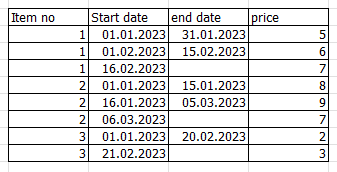1 ACCEPTED SOLUTION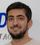Solution Sage

Sorry. I did not check the total that is not correct.

After omitting the relationship between your DateTable and StartDate,

You can fix it with :

1-Create a measure:

SalesQuantityNew=

SUM(ItemSales[Quantity])

Then Change your NewSales as below:

NewSales =
SUMX (
DateTable,
SUMX (
FILTER (
ItemPrice,
ItemPrice[StartDate] <= DateTable[Date]
&& ItemPrice[NewEndDate] >= DateTable[Date]
),
[SalesQuantityNew] * 'ItemPrice'[Price]
)
)

If this is your answer, please mark it as an accepted solution so others can find it easily between the long discussions that happened.

Regards,
Loran
17 REPLIES 17Helper I

@MohammadLoran25 Thank you so much for taking your time on this one.

It seems like i get the amount on every entry-date. But wat i want is total quantity pr item (regardless of entry-date) * price on the spesific entry-date.  Should end up something like this:

Totals:

Yellow = total quantity on all entries.

Green = price * total quantity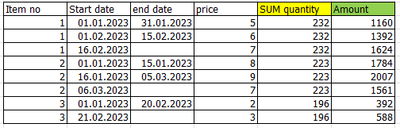Final desired output: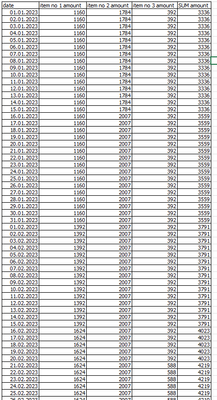Solution Sage

Okay. Then it would be easier.

You do not need any calculated columns.

Just create these 2 measures:

1-

SalesQuantity =
VAR _ITEM =
SELECTEDVALUE ( ItemPrice[ItemNo] )
RETURN
CALCULATE (
SUM ( ItemSales[Quantity] ),
FILTER ( ItemSales, ItemSales[ItemNo] = _ITEM )
)

2-

SalesAmount =
VAR _PRICE = SELECTEDVALUE ( ItemPrice[Price] ) RETURN _PRICE * [SalesQuantity]

If this answer solves your problem, please mark it as an accepted solution so the others would find what they need easier.
Regards,
LoranHelper I

Thank you!

We are almost there. The SalesAmount only seem to work when filter on each product.

Is it any way to sum the total amount on all 3 items?

As it is in the table above in column named "SUM Amount"Solution Sage

You're welcome.

Yes, if you want it as a column you can make a calculated column as below:

SUM Quantity=[SalesQuantity]

[SalesQuantity] It is the measure in my previous message.

To get total amount on all 3 items, you can change the previous measure as below:

SalesAmount=

SUMX(ItemPrice, 'ItemPrice'[SUM Quantity]*'ItemPrice'[Price])Helper I

I want the sales amount for all items on each and every date. The measures seems to give me the amount on the specific StartDate. For example 16.02.2023 gives me the numer 1624. But it should give me (item1: 1624 + item2: 2007+ item 3: 392= ) 4.023Solution Sage

Oh man,

What a long discussion. LOLL

Finally I think I got what you need.

Assume your date table name is DateTable and Check the measure below:

NewSalesMeasure =
SUMX (
DateTable,
SUMX (
FILTER (
ItemPrice,
ItemPrice[StartDate] <= DateTable[Date]
&& ItemPrice[NewEndDate] >= DateTable[Date]
),
SUMX ( ItemPrice, [SalesQuantity] * 'ItemPrice'[Price] )
)
)

Then by putting date from your DateTable and ItemNo and This measure you can get what you need.Helper I

Thank you.

I have set a relationship between Date and StartDate.

As you could see there is no data on for example 17.02.2023. And the 16.02.2023 only has 1624, witch is the StartDate for item 1. It should be amount on the other two items, and the total sum.

Top table has "Show items with no data" on. (Date), the bottom table is off.Solution Sage

Because you want the measure per each date, so you should write the measure on a separate datetable and only filter the datetable like what I did in my newmeasure.

You can make a new datetable with no relationahip or use CROSSFILTER in the measure to deactivate the relationship in the context of measure.Solution Sage

In my measure, you should delete the relationship betweem startdate and your datetable. Then it works. Check it.Helper I

This is what i get without any relationships: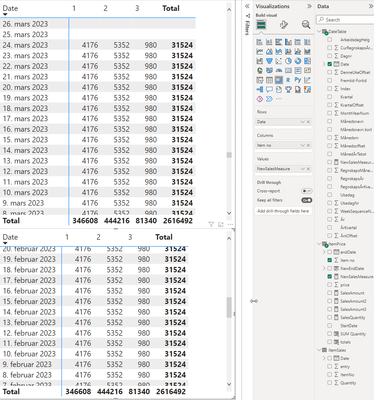Solution Sage

Sorry. I did not check the total that is not correct.

After omitting the relationship between your DateTable and StartDate,

You can fix it with :

1-Create a measure:

SalesQuantityNew=

SUM(ItemSales[Quantity])

Then Change your NewSales as below:

NewSales =
SUMX (
DateTable,
SUMX (
FILTER (
ItemPrice,
ItemPrice[StartDate] <= DateTable[Date]
&& ItemPrice[NewEndDate] >= DateTable[Date]
),
[SalesQuantityNew] * 'ItemPrice'[Price]
)
)

If this is your answer, please mark it as an accepted solution so others can find it easily between the long discussions that happened.

Regards,
LoranHelper I

I changed the starting date on price for item #3, the earliest date is now 01.02.23.

My visual looks now like this: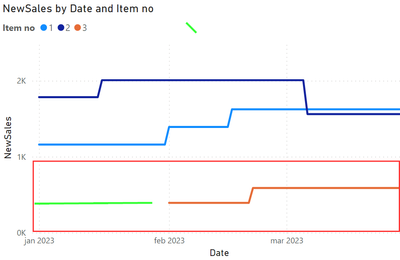As there is no price from 01.01.23 to 31.01.23 there is nothing to show. Even though there is sales data.

I want to use the price starting from 01.02.23 for sales in the dateperiode 01.01.23 -> 31.01.23. (see green line)

Something like: If SalesDate < earliest StartDate (pr item), use earliest StartDate. Else < startDate && >EndDateSolution Sage

Hi @Wikolin ,
What a good question !

1-Create a measure as below:

MinStartDateMeasure =
MINX (
FILTER ( ALL ( ItemPrice ), ItemPrice[ItemNo] = MIN ( ItemPrice[ItemNo] ) ),
ItemPrice[StartDate]
)

2-Then Change your NewSales Measure to the this one:

NewSales =
SWITCH (
TRUE (),
SUMX (
FILTER (
DateTable,
DateTable[Date]
<= CALCULATE ( MAX ( ItemPrice[NewEndDate] )ALL ( ItemPrice ) )
),
SUMX (
FILTER (
ItemPrice,
ItemPrice[StartDate] <= DateTable[Date]
&& ItemPrice[NewEndDate] >= DateTable[Date]
),
1
)
) > 0,
SUMX (
FILTER (
DateTable,
DateTable[Date]
<= CALCULATE ( MAX ( ItemPrice[NewEndDate] )ALL ( ItemPrice ) )
),
SUMX (
FILTER (
ItemPrice,
ItemPrice[StartDate] <= DateTable[Date]
&& ItemPrice[NewEndDate] >= DateTable[Date]
),
[SalesQuantity] * 'ItemPrice'[Price]
)
),
SUMX (
FILTER (
DateTable,
DateTable[Date]
<= CALCULATE ( MAX ( ItemPrice[NewEndDate] )ALL ( ItemPrice ) )
),
SUMX (
FILTER (
ItemPrice,
ItemPrice[ItemNo] = MIN ( ItemPrice[ItemNo] )
&& ItemPrice[StartDate] = [MinStartDatemEASURE]
),
[SalesQuantity] * 'ItemPrice'[Price]
)
)
)

If this answer solves your problem, please give it a thumbs up and mark it as an accepted solution so the others would find what they need easier.

Regards,
LoranHelper I

It worked in one case, but the total does not change.

As you see here, the calculations on each item is the same on each date in this periode.

But the total changes from 01.02.23. (because the real price starts then on item #3)

Would like the total to be (1392+2007+392 = ) 3791.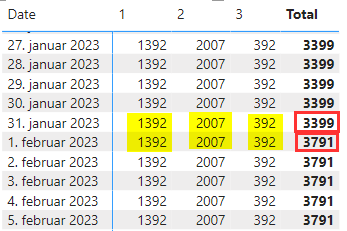Solution Sage

Just need to create a measure based on the last measure we created:

NewMeasureWithCorrectTotal=

SUMX(VALUES(ItemPrice[ItemNo]),[NewSales])

**Please keep in mind that because of using SUMX in several measures, the measure might be slow. So based on your data size, if there is any performance problem, of course you need to revise the measures accordingly. Now the logic is clear so changing it would be easier.

Regards,Helper I

This works perfectly. A minor adjustment. I used [SalesQuantity] instead of [SalesQuantityNew]. Thank you so much @MohammadLoran25Solution Sage

Hi @Wikolin ,

Assume the name of your first table is ItemPrice

the name of your second table is ItemSales.

1-In your ItemPrice table, Create a calculated column as below:

NewEndDate = IF(ISBLANK(ItemPrice[EndDate]),TODAY(),ItemPrice[EndDate])

2-In your ItemSales table, Create a calculated column as :

PriceBasedOnDate =
CALCULATE (
MIN ( ItemPrice[Price] ),
FILTER (
ItemPrice,
ItemPrice[ItemNo] = ItemSales[ItemNo]
&& ItemSales[Date] >= ItemPrice[StartDate]
&& ItemSales[Date] <= ItemPrice[NewENDDate]
)
)

3-Your sales would be a measure like:

Amount=
SUMX('ItemSales',
'ItemSales'[Quantity]*'ItemSales'[PriceBasedOnDate]
)

If this answer solves your problem, please mark it as an accepted solution so the others would find what they need easier.
Regards,
Loran